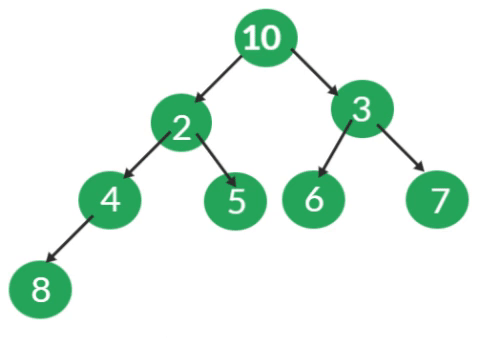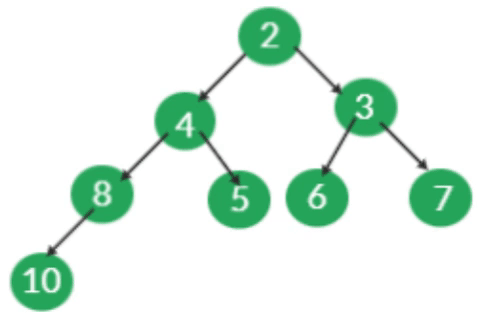# Binary Heap Heapify and Extract Min

Problem statement :

Given a Binary Heap, Implement Hepify() and ExtractMin() operations on Binary Heap.

Disclaimer: Don’t jump directly to the solution, try it out yourself first.

### Heapify():

• Suppose there exists a Node at some index i, where the Minheap property is Violated.
• We assume that all the subtrees of the tree rooted at index i are valid binary heaps.
• The Heapify function is used to restore the Minheap, by fixing the violation.

Steps to be followed for Heapify:

• First find out the Minimum among the Violated Node, left, and right child of Violated Node.
• If the Minimum among them is the left child, then swap the Violated Node value with the Left child value and recursively call the function on the left Child.
• If the Minimum among them is the right child, then swap the Violated Node value with the right child value and recursively call the function right Child.
• Recursive call stops when the heap property is not violated.### ExtractMin():

• This removes the Minimum element from the heap.

Steps to be followed to Remove Minimum value/root Node:

• Copy the last Node value to the Root Node, and decrease the size, this means that the root value is now deleted from the heap, and the size is decreased by 1.
• By doing the above step we ensure that the Complete binary tree property is not violated, as we are copying the last node value to the root node value, the Min Heap property gets violated.
• Call the Heapify function to convert it into a valid heap.Code:

## C++ Code

``````#include <bits/stdc++.h>

using namespace std;

class BinaryHeap {
public:
int capacity; /*Maximum elements that can be stored in heap*/
int size; /*Current no of elements in heap*/
int * arr; /*array for storing the keys*/

BinaryHeap(int cap) {
capacity = cap; /*Assigning the capacity*/
size = 0; /*Intailly size of hepa is zero*/
arr = new int[capacity]; /*Creating a array*/
}

/*returns the parent of ith Node*/
int parent(int i) {
return (i - 1) / 2;
}
/*returns the left child of ith Node*/
int left(int i) {
return 2 * i + 1;
}
/*Returns the right child of the ith Node*/
int right(int i) {
return 2 * i + 2;
}

/*Insert a new key x*/
void Insert(int x) {
if (size == capacity) {
cout << "Binary Heap Overflwon" << endl;
return;
}
arr[size] = x; /*Insert new element at end*/
int k = size; /*store the index ,for checking heap property*/
size++; /*Increase the size*/

/*Fix the min heap property*/
while (k != 0 && arr[parent(k)] > arr[k]) {
swap( & arr[parent(k)], & arr[k]);
k = parent(k);
}
}

void Heapify(int ind) {
int ri = right(ind); /*right child*/
int li = left(ind); /*left child*/
int smallest = ind; /*intially assume violated value in Min value*/
if (li < size && arr[li] < arr[smallest])
smallest = li;
if (ri < size && arr[ri] < arr[smallest])
smallest = ri;
/*smallest will store the minvalue index*/
/*If the Minimum among the three nodes is the parent itself,
that is Heap property satisfied so stop else call function recursively on Minvalue node*/
if (smallest != ind) {
swap( & arr[ind], & arr[smallest]);
Heapify(smallest);
}
}
int getMin() {
return arr;
}
int ExtractMin() {
if (size <= 0)
return INT_MAX;
if (size == 1) {
size--;
return arr;
}
int mini = arr;
arr = arr[size - 1]; /*Copy last Node value to root Node value*/
size--;
Heapify(0); /*Call heapify on root node*/
return mini;
}
void swap(int * x, int * y) {
int temp = * x;
* x = * y;
* y = temp;
}
};
int main()

{
BinaryHeap h(20);
h.Insert(4);
h.Insert(1);
h.Insert(2);
h.Insert(6);
h.Insert(7);
h.Insert(3);
h.Insert(8);
h.Insert(5);
cout << "Min value is " << h.getMin() << endl;
h.Insert(-1);
cout << "Min value is " << h.getMin() << endl;
h.ExtractMin();
cout << "Min value is " << h.getMin() << endl;
return 0;
}``````

Output:

Min value is 1
Min value is -1
Min value is 1

Time Complexities :

Heapify()  – O(logN)
ExtractMin() – O(logN)

## Java Code

``````import java.util.*;

class BinaryHeap {
static int capacity; /*Maximum elements that can be stored in heap*/
static int size; /*Current no of elements in heap*/
static int arr[]; /*array for storing the keys*/

BinaryHeap(int cap) {
capacity = cap; /*Assigning the capacity*/
size = 0; /*Intailly size of hepa is zero*/
arr = new int[capacity]; /*Creating a array*/
}

/*returns the parent of ith Node*/
static int parent(int i) {
return (i - 1) / 2;
}
/*returns the left child of ith Node*/
static int left(int i) {
return 2 * i + 1;
}
/*Returns the right child of the ith Node*/
static int right(int i) {
return 2 * i + 2;
}

/*Insert a new key x*/
static void Insert(int x) {
if (size == capacity) {
System.out.println("Binary Heap Overflown");
return;
}
arr[size] = x; /*Insert new element at end*/
int k = size; /*store the index ,for checking heap property*/
size++; /*Increase the size*/

/*Fix the min heap property*/
while (k != 0 && arr[parent(k)] > arr[k]) {
int temp = arr[parent(k)];
arr[parent(k)] = arr[k];
arr[k] = temp;
k = parent(k);
}
}

static void Heapify(int ind) {
int ri = right(ind); /*right child*/
int li = left(ind); /*left child*/
int smallest = ind; /*intially assume violated value in Min value*/
if (li < size && arr[li] < arr[smallest])
smallest = li;
if (ri < size && arr[ri] < arr[smallest])
smallest = ri;
/*smallest will store the minvalue index*/
/*If the Minimum among the three nodes is the parent itself,
that is Heap property satisfied so stop else call function recursively on Minvalue node*/
if (smallest != ind) {
int temp = arr[ind];
arr[ind] = arr[smallest];
arr[smallest] = temp;
Heapify(smallest);
}
}
static int getMin() {
return arr;
}
static int ExtractMin() {
if (size <= 0)
return Integer.MAX_VALUE;
if (size == 1) {
size--;
return arr;
}
int mini = arr;
arr = arr[size - 1]; /*Copy last Node value to root Node value*/
size--;
Heapify(0); /*Call heapify on root node*/
return mini;
}

public static void main(String args[])

{
BinaryHeap h = new BinaryHeap(20);
h.Insert(4);
h.Insert(1);
h.Insert(2);
h.Insert(6);
h.Insert(7);
h.Insert(3);
h.Insert(8);
h.Insert(5);
System.out.println("Min value is " + h.getMin());
h.Insert(-1);
System.out.println("Min value is " + h.getMin());
h.ExtractMin();
System.out.println("Min value is " + h.getMin());

}
}``````

Output:

Min value is 1
Min value is -1
Min value is 1

Time Complexities :

Heapify()  – O(logN)
ExtractMin() – O(logN)